Next: Interferometer Up: 30m Telescope Previous: Successful 19--channel Bolometer

## Conversion of source velocity/redshift to sky frequency

Sometimes confusion may arise on how to calculate the sky frequency for a given redshift or source velocity. This stems from the fact that optical and radio astronomers calculate radial source velocities from different formulas. (Remember that velocities are not directly measured but calculated from wavelength or frequency shifts). Optical astronomers deduce radial velocities from the measurement of a wavelength shift: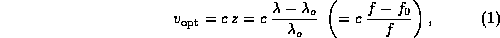where c is the speed of light,(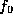) the unshifted and(f) the shifted wavelength (frequency), respectively. The astronomical redshift z is defined as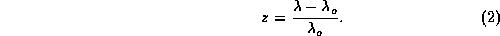At low redshifts some radio astronomers use a different convention which presumably comes from the fact that frequency shifts rather than wavelength shifts are measured. The radio convention is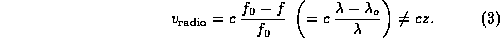As a consequence, radio ``velocity'' and optical recession velocity are not the same for a given redshift: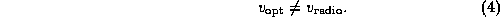This means that in order to calculate the correct sky frequency from a given velocity one needs to know if the velocity was calculated using the optical or radio convention. An optical velocity(or redshift z) requires another formula than a radio velocityobtained e.g. from 21 cm HI measurements. The sky frequency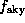for a given source velocity and line rest frequencyis

• optical convention: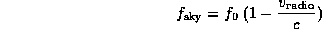For a given velocity the sky frequencies calculated with the two formulas above can differ significantly. For example, calculating the sky frequency in the 230 GHz range with the inappropriate formula (optical instead of radio convention, or vice versa) at a source velocity of 10000 km/sec results in an error of about 250 MHz. At 20000 km/sec, this error has increased to about 1 GHz!

At the IRAM 30m telescope the conversion is done in the following way: the drive program calculates the velocity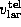of the telescope relative to the local standard of rest, takes the source velocity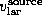(from the source catalogue), and then calculates the sky frequency applying the optical convention: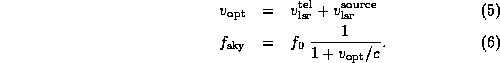If you want to observe e.g. a high-redshift object with redshift z at line rest frequency, the recommended procedure is to set the source velocity v (in the source catalogue) to zero and give the corrected frequency (calculated with the optical formula)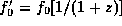to the drive program by using the OBS command:
RECEIVER rx_name /FREQUENCYLSB.

You might alternatively calculate the optical source velocity, give this velocity in the source catalogue, and use the line rest frequencyin the OBS command:
RECEIVER rx_name /FREQUENCYLSB.

If you need to use the radio convention, it is best to set the source velocity v (in the source catalogue) to zero and give the corrected frequency (calculated with the radio formula)using the OBS command:
RECEIVER rx_name /FREQUENCY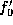LSB
instead of the line rest frequency.

Instead of giving frequencies directly in the RECEIVER command, you can also define a line with the corrected frequency in your line catalogue, and use the OBS command: